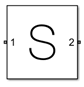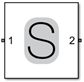# S-Parameters

Model S-parameter network

•Libraries:
RF Blockset / Circuit Envelope / Elements

## Description

The S-parameters block models a network defined by S-parameters in the RF Blockset™ circuit envelope simulation environment. The device can have up to 65 ports. For an introduction to RF simulation, see the example, Simulate High Frequency Components.

Note

If you specify more than eight ports, the block does not simulate noise.

The block models S-parameter data in the RF Blockset environment by fitting a rational function to the specified data. For more information about rational fitting of S-parameters, see the RF Toolbox™ `rational` function.

Note

During the fitting procedure, the block sets the `TendsToZero` and `Qlimit` properties of the `rational` object to `false` and `inf`, respectively.

S-parameters block mask icons are dynamic and indicate the current state of the applied noise parameter. This table shows you how the icons on this block vary based on the state of the Simulate noise parameter on the block.

Simulate noise: `on`Simulate noise: `off`To model RF complex baseband S-parameters in Simulink, use the Idealized Baseband S-parameters block.

## Parameters

expand all

Main

Data source for S-parameters behavior, specified as one of the following:

• `Data file` — Name of a Touchstone file with the extension`.s2p`. The block ignores noise and nonlinearity data in imported files.

• `Network-parameters` — Provide Network parameter data such as `S-parameters`, `Y-parameters`, and `Z-parameters` with corresponding Frequency and Reference impedance (ohms) for the s-parameters.

• `Rational model` — Provide values for Residues, Poles, and Direct feedthrough parameters which correspond to the equation for a rational model

`$F\left(s\right)=\left(\sum _{k=1}^{n}\frac{{C}_{k}}{s-{A}_{k}}+D\right)\begin{array}{cc},& s=j2\pi f\end{array}$`

.In this rational model equation, each Ck is the residue of the pole Ak. If Ck is complex, a corresponding complex conjugate pole and residue must also be enumerated. This object has the properties `C`, `A`, and `D`. You can use these properties to specify the Residues, Poles, and Direct feedthrough parameters.

Name of network parameter data file, specified as a character vector.

#### Dependencies

To enable this parameter, select `Data file` in Data source tab.

Select this parameter to use a network parameter object as the data source to model the S-parameters.

Once you select this parameter, in the text box that opens, specify one of these:

• Network parameter object.

• MATLAB® base workspace variable or a Simulink® model workspace variable. The block evaluates if the specified variable is a network parameter object.

`sparameters('default.s2p')` is the default network parameter object when you select this parameter.

The network parameter objects that you can specify include:

#### Dependencies

To enable this parameter, set Data source to `Network-parameters`.

Network parameter type, specified as `S-parameters`, `Y-parameters`, or `Z-parameters`.

#### Dependencies

To enable this parameter, set Data source to `Network-parameters`.

Network parameter values specified as a multidimensional array or a scalar. When you specify Network parameters as a multi-dimensional array, the third dimension of the S-parameter array must be of the same length as the frequencies vector specified in the Frequency (dB) parameter.

#### Dependencies

To enable this parameter, set Data source to `Network-parameters`.

Frequency of network parameters, specified as a scalar or a vector in Hz.

#### Dependencies

To enable this parameter, set Data source to `Network-parameters`.

Reference impedance of network parameters, specified as a scalar.

#### Dependencies

To enable this parameter, set Data source to `Network-parameters`.

Select this parameter to use a `rational` object as the data source to model S-parameter.

Once you select this parameter, in the text box that opens, specify one of these:

• `rational` object.

• MATLAB base workspace variable or a Simulink model workspace variable. The block evaluates if the specified variable is a rational object."

`rational('default.s2p')` is the default `rational` object when you select this parameter.

The `rational` objects that you can specify include:

#### Dependencies

To enable this parameter, set Data source to `Rational model`.

Residues in order of rational model, specified as a vector.

#### Dependencies

To enable this parameter, set Data source to `Rational model`.

Poles in order of rational model, specified as a vector.

#### Dependencies

To enable this parameter, set Data source to `Rational model`.

Direct feedthrough, specified as an array vector.

#### Dependencies

To enable this parameter, set Data source to `Rational model`.

Choose this parameter to generate thermal noise waves . Clear this parameter to stop simulating noise. For more information see, Generate Thermal Noise.

Note

This parameter is cleared when you specify more than eight ports.

Select this parameter to ground and hide the negative terminals. Clear this parameter to expose the negative terminals. By exposing these terminals, you can connect them to other parts of your model.

Modeling

Model S-parameters, specified as:

• `Time domain (rationalfit)` technique creates an analytical rational model that approximates the whole range of the data. When modeling using ```Time domain```, the in `Visualization` tab plots the data defined in `Data Source` and the values in the `rational` function.

• `Frequency domain`computes the baseband impulse response for each carrier frequency independently. This technique is based on convolution. There is an option to specify the duration of the impulse response. For more information, see Compare Time and Frequency Domain Simulation Options for S-parameters.

• For the Amplifier, Antenna, and S-parameters blocks, the default value is ```Time domain (rationalfit)```. For the Transmission Line block, the default value is `Frequency domain`.

#### Dependencies

To enable this parameter, set Data source to either ```Data file``` or `Network-parameters`.

Rational fit fitting options, specified as ```Fit individually```, ```Share poles by column```, or ```Share all poles```.

Rational fitting results shows values of Number of independent fits, Number of required poles, and Relative error achieved (dB).

#### Dependencies

To set this parameter, select ```Time domain (rationalfit)``` in Modeling options.

Relative error acceptable for the rational fit, specified as a scalar.

#### Dependencies

To set this parameter, select `Time domain (rationalfit)` in Modeling options.

Maximum number of poles to control over the fitting procedure, specified as a positive scalar. You can specify up to `99` poles.

#### Dependencies

To set this parameter, select ```Time domain (rationalfit)``` in Modeling options.

Select this parameter to automatically calculate impulse response. Clear this parameter to manually specify the impulse response duration using Impulse response duration.

#### Dependencies

To set this parameter, select `Frequency domain` in Modeling options.

Impulse response duration, specified as a scalar.

#### Dependencies

To set this parameter, first select `Frequency domain` in Modeling options. Then, clear ```Automatically estimate impulse response duration```.

Select this parameter to ignore the S-parameter phase and to delay the impulse response by half its length. You can use this parameter to shape spectral content with filter effects by specifying only magnitude.

This parameter uses the magnitude of the original data to obtain the impulse response of the block. This impulse response is centered about the time sequence and adds an inherent delay of half the duration of the impulse response. In the frequency domain, this translates to a linear monotonic decrease in phase throughout the given spectrum.

Note

• This parameter is applicable only for S-parameter data modeled in the frequency domain and introduces an artificial delay to the system.

• Adding a time delay (or frequency phase variation) to the system can result in unexpected behaviors. For example, in an unmatched system, delayed signals are bounced back and added to the original signals.

#### Dependencies

To enable this parameter, set Modeling options to `Frequency domain`.

Visualization

Frequency data source, specified as:

When Source of frequency data is ```Extracted from data source```, the Data source must be set to `Data file`. Verify that the specified Data file contains frequency data.

When Source of frequency data is `User-specified`, specify a vector of frequencies in the Frequency data parameter. Also, specify units from the corresponding drop-down list.

Frequency data range, specified as a vector or a scalar in Hz, kHz, MHZ, or GHz. Specify Frequency data as a scalar when you want to visualize a singe frequency S-parameter data.

#### Dependencies

To enable this parameter, set Source of frequency data to `User-specified`.

Type of data plot that you want to produce with your data specified as one of the following:

• `X-Y plane` — Generate a Cartesian plot of your data versus frequency. To create linear, semilog, or log-log plots, set the Y-axis scale and X-axis scale accordingly.

• `Polar plane` — Generate a polar plot of your data. The block plots only the range of data corresponding to the specified frequencies.

• `Z smith chart`, ```Y smith chart```, and `ZY smith chart` — Generate a Smith® chart. The block plots only the range of data corresponding to the specified frequencies.

Type of S-Parameters to plot, specified as `SNN`, where N is the number of ports in the s-parameters block.

Type of S-Parameters to plot, specified as `SNN`, where N is the number of ports in the s-parameters block.

Plot format, specified as `Magnitude (decibels)`, `Angle(degrees)`, `Real`, or `Imaginary`.

Plot format, specified as `Magnitude (decibels)`, `Angle(degrees)`, `Real`, or `Imaginary`.

Y-axis scale, specified as `Linear` or `Logarithmic`.

X-axis scale, specified as `Linear` or `Logarithmic`.

Plot specified data using plot button.